## How to Calculate Net Present Value (Npv) in Excel

In this tutorial, you will learn to calculate Net Present Value, or NPV, in Excel.

Net Present Value is a financial function that is calculated for an investment, and it represents the present value of the investment minus the amount of money that costs to buy in. Excel offers a preset function for this called NPV.

Please be aware that all the investment cash flows must occur at the same interval for the calculation to be accurate.

NPV has two arguments: rate – which refers to the discount rate, and the range of values that contains future cash flows.

Step 1: Open the document in which you want to calculate NPV.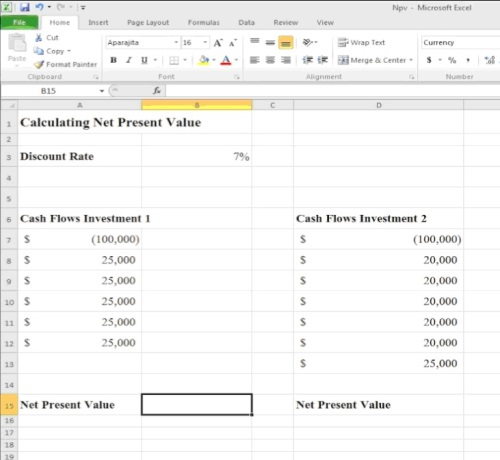Step 2. Go to the cell where you want the function to be calculated, and type the following:
= npv (our discount rate /12 as the rate is compounded monthly, the range of values you want to be considered)+the initial investment, in our case the starting 100,000\$.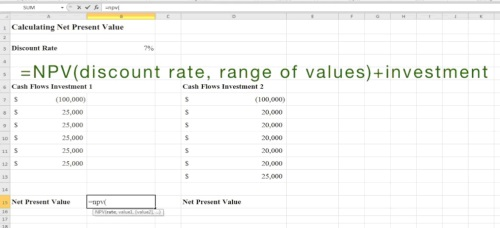Step 3. Excel will calculate for you the Net Present Value of this investment.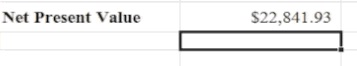Step 4. Go to the cell that you want to hold the NPV result for comparison, and type:
=npv(the same discount rate/12,the range of values)+the initial investment. Hit Enter.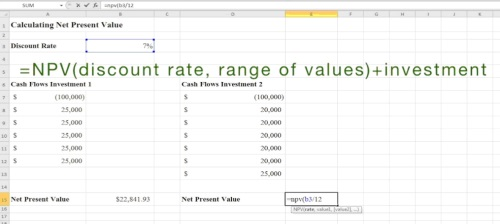Step 5. Excel will calculate for you the Net Present Value of this investment.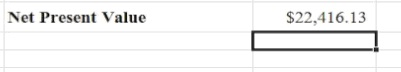Step 6. Now that we see both results, we will agree that the first option is better and proceed with it.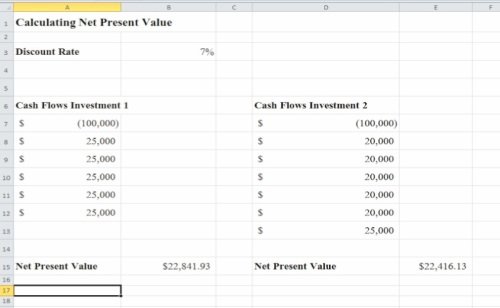Result: Congratulations, you have learned how to calculate the Net Present Value in Excel.